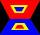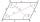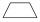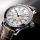# Angles 1

It is true neighboring angles have not common arm?

Result

Leave us a comment of example and its solution (i.e. if it is still somewhat unclear...):

Showing 0 comments:Be the first to comment!## Next similar examples:

1. Rhombus anglesIf one angle in the rhombus is 159°, what is it neighboring angle in rhombus?
2. Rectangular trapezoidHow many inner right angles has a rectangular trapezoid?
3. ParallelogramIn the parallelogram we know one internal angle 67°33`. Calculate the other internal angles.
4. Isosceles trapeziumTrapezoid YSED (YS||ED) is isosceles. The size of the angle at vertex Y is 17 degrees. Calculate the size of the angle at vertex E.
5. Minute angleDetermine size of angle, which takes minute hand for 75 minutes.
6. Fraction and a decimalWrite as a fraction and a decimal. One and two plus three and five hundredths
7. Addition of Roman numbersAdded together and write as decimal number: LXVII + MLXIV
8. Bus 14Boatesville is 65.35 kilometers from Stanton. A bus traveling from Stanton is 24.13 kilometers from Boatesville. How far has the bus traveled?
9. In fractionsAn ant climbs 2/5 of the pole on the first hour and climbs 1/4 of the pole on the next hour. What part of the pole does the ant climb in two hours?
10. ExpressionSolve for a specified variable: P=a+4b+3c, for a
11. Fraction to decimalWrite the fraction 3/22 as a decimal.
12. ZdeněkZdeněk picked up 15 l of water from a 100-liter full-water barrel. Write a fraction of what part of Zdeněk's water he picked.
13. LineCan we construct a line segment, if we know: center
14. SimplifySimplify the following problem and express as a decimal: 5.68-[5-(2.69+5.65-3.89) /0.5]
15. Forest nurseryIn the forest nursery after winter, they found that 1/10 stems died out of them. For them, they land 193 new spruces. How many spruces are in the forest nursery?
16. Lengths of the poolMiguel swam 6 lengths of the pool. Mat swam 3 times as far as Miguel. Lionel swam 1/3 as far as Miguel. How many lengths did mat swim?
17. Mixed2improperWrite the mixed number as an improper fraction. 166 2/3